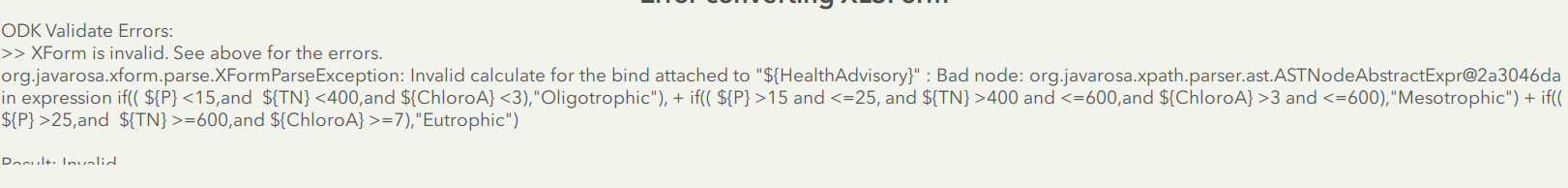# How to calculate a field based on multiple conditions?

354
5
04-02-2019 07:51 AM
Highlighted

I am creating a survey that is going to be used to collect water quality. The end user has specific criteria that they would like to use in symbolizing the data. The specific criteria are:Basically, if the answers to 3 questions  meet one of those criteria, I want to calculate a separate field to auto-fill the appropriate Description, and use that to symbolize the data. Currently I have this in the calculation field:

if((\${P}<15,and \${TN}<400,and\${ChloroA}<3),"Oligotrophic"), +  if((\${P}>15 and <=25, and\${TN}>400 and <=600,and\${ChloroA}>3 and <=600),"Mesotrophic") + if((\${P}>25,and \${TN}>=600,and\${ChloroA}>=7),"Eutrophic")

I've only recently taken the deep dive into creating more complex surveys so I could be really missing something here. I have attached a copy of my survey sheet for reference.

EDIT:

Here is the error I keep receiving:Tags (2)
5 Replies
Highlighted
byEsri Frequent Contributor

Hi Michael,

You can not mix and match the if statements with mathematical operator such as + sign. You need to use nested if statements or re-write your expressions to use AND operators and put brackets around each part in your expression.

Phil.

Highlighted

So I've seen the nested if-statement suggested multiple times and have now moved to this:

if(\${P}<15 and \${TN}<400 and \${ChloroA}<3),"Oligotrophic", if(\${P}>15 and <=25, and \${TN}>400 and <=600 and \${ChloroA}>3 and <=600),"Mesotrophic", if(\${P}>25 and \${TN}>=600 and \${ChloroA}>=7),"Eutrophic")

However, I'm still not having any success. I think my parenthesis are off, but cannot figure out how...

Highlighted
byEsri Esteemed Contributor

Hi Michael,

The if() statements need to be nested within each other.  For example, if you wanted to determine which school type a student was in by grade (1-6 Elementary, 7-8 Middle, 9-12 High), we can break this into 2 if statements:

1) is the student in an elementary school

2), if 1 is no, is the student in middle school

If 2) is no, we know they are in high school.  This gets implemented as

`if(\${grade} <=6, "Elementary", if(\${grade} <=8, "Middle", "High"))`

Note that at the right end there are 2 right parenthesis - one for each if() statement.  Also note that I can remove the check in the second if() for grades 1-6 - we already addressed that in the first if() statement.

In the formula you have above, you have the evaluation criteria (for example,  \${P}<15 and \${TN}<400 and \${ChloroA}<3 ), in parenthesis by itself - the true and false values also need to be inside the parenthesis as well;

`if(\${P}<15 and \${TN}<400 and \${ChloroA}<3),"Oligotrophic", <Mesotrophic/Eutrophic IF>)`

would be the first, with the next if statement in the <Mesotrophic/Eutrophic IF> area.

Highlighted
byEsri Esteemed Contributor

Hi Michael,

This is the same question as you brought up in https://community.esri.com/thread/210714-if-statement-calculation-survey123#comment-842469 , yes?

Highlighted

Yes, I first asked as a comment, and then thought it'd be better just to make my own post. I am trying your solution this morning, but also got a comment regarding "nested" if statements, so I'm going to try that too.